﻿ 敏捷卫星总体参数对像质的影响分析

# 敏捷卫星总体参数对像质的影响分析Analyzing the Influence of the Overall Parameters of Agile Satellite on the Image Quality

Abstract: In order to meet the fast response of remote sensing satellite and the requirement of high-resolution imaging, agile satellites with dexterity and efficiency become the focus of research. For TDI-CCD sensors, the image shift caused by agile satellites with fast mobility is harmful for high quality imaging. According to the different attitude angles, the corresponding map of star-Land and the model of the velocity of the image shift are established. The influence of satellite parameters such as orbital altitude and attitude angle on the image moving velocity and bias angle is analyzed, which is very important for the imaging quality of the camera.

1. 引言

2. 卫星总体参数对像移的影响分析

2.1. 星下点成像模式下的像移速度

2.1.1. 星下点成像模型

2.1.2. 轨道运动产生的像移速度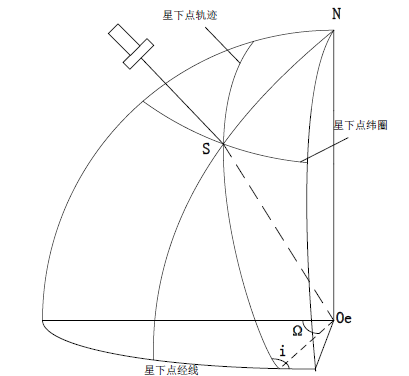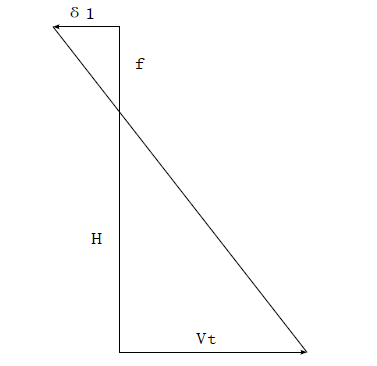Figure 2. Principle diagram of orbit motion image shift

${V}_{1}=f\cdot \frac{{V}_{g}}{L}$ (1)

2.1.3. 地球自转产生的像移速度

${V}_{2}=\frac{f}{L}\cdot {\omega }_{e}\cdot \left(R+H\right)\cdot \mathrm{cos}{\delta }_{s}$ (2)

2.2. 侧摆姿态机动成像模型

2.2.1. 轨道运动产生的像移速度

${V}_{1}=f\cdot \frac{V}{L}=f\cdot \frac{{\Omega }_{o}R\mathrm{cos}b}{L}$ (3)

2.2.2. 地球自转产生的像移速度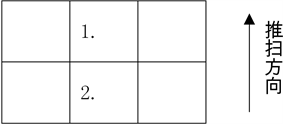Figure 3. Diagram of image shift directionFigure 4. Diagram of image shift direction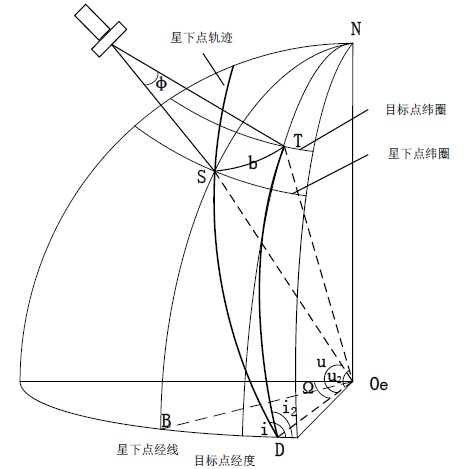Figure 5. Roll attitude imaging

$\angle NST$ 取值不同。根据球面三角形相关公式求得：

${\delta }_{T}=\mathrm{arcsin}\left(\mathrm{sin}{\delta }_{s}\mathrm{cos}b±\mathrm{cos}{\delta }_{s}\mathrm{sin}b\mathrm{cos}i\right)$ (4)

$u=\mathrm{arcsin}\left(\frac{\mathrm{sin}\delta }{\mathrm{sin}i}\right)$ (5)

${u}_{2}=\mathrm{arccos}\left(\mathrm{cos}u\mathrm{cos}b\right)$ (6)

${i}_{2}=i-\mathrm{arccos}\left(\mathrm{tan}u\mathrm{cot}{u}_{2}\right)$ (7)

${V}_{2}=\frac{f}{L}\cdot {\omega }_{e}\cdot R\cdot \mathrm{cos}{\delta }_{T}$ (8)

2.3. 俯仰姿态机动后成像模型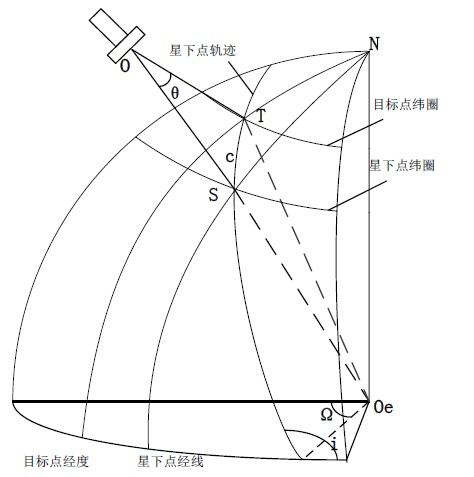Figure 6. Pitch attitude imaging

2.3.1. 轨道运动产生的像移速度

${V}_{1}=f\cdot \frac{{\Omega }_{o}R}{L}$ (9)

2.3.2 地球自转产生的像移速度

${\delta }_{T}=\mathrm{arcsin}\left(\mathrm{sin}{\delta }_{s}\mathrm{cos}c±\mathrm{cos}{\delta }_{s}\mathrm{sin}c\mathrm{sin}i\right)$ (10)

c在 $\Delta O{O}_{e}T$ 利用正弦定理求得，

$c=\angle S{O}_{e}T=\mathrm{arcsin}\left(\frac{R+H}{R}\mathrm{sin}\theta \right)-\theta$ (11)

${V}_{2}=f\cdot \frac{R\cdot {\omega }_{e}\cdot \mathrm{cos}{\delta }_{T}}{L}$ (12)

2.4. 侧摆加俯仰姿态机动后成像模型

2.4.1. 轨道运动产生的像移速度

$b=\angle S{O}_{e}T=\mathrm{arcsin}\left(\frac{{R}_{s}}{{R}_{e}}\mathrm{sin}\varphi \right)-\varphi$ (13)

$V={\Omega }_{o}\left(R+H\right)\mathrm{cos}b$ (14)

2.4.2. 地球自转产生的像移速度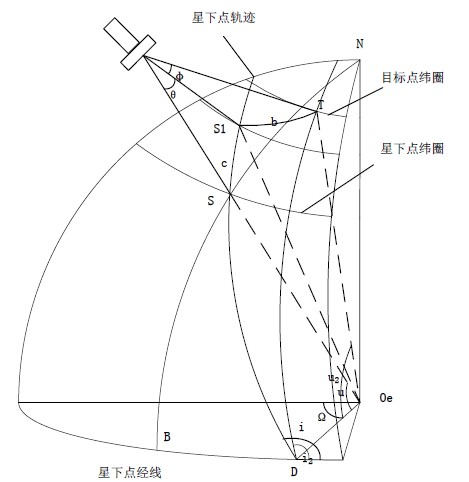Figure 7. Pitch and roll imaging

$u=\mathrm{arcsin}\left(\frac{\mathrm{sin}{\delta }_{s}}{\mathrm{sin}i}\right)$ (15)

$\beta =\mathrm{arcsin}\left(\frac{R+H}{H}\mathrm{sin}\theta \right)-\theta$ (16)

${u}_{1}=u+\beta$ (17)

 (18)

${u}_{2}=\mathrm{arccos}\left(\mathrm{cos}{u}_{1}\mathrm{cos}n\right)$ (19)

${i}_{2}=i-\mathrm{arccos}\left(\mathrm{tan}{u}_{1}\mathrm{cot}{u}_{2}\right)$ (20)

${\delta }_{T}=\mathrm{arcsin}\left(\mathrm{sin}{u}_{2}\mathrm{sin}{i}_{2}\right)$ (21)

${V}_{e}={\Omega }_{e}\left(R+H\right)\mathrm{cos}{\delta }_{T}$ (22)

3. 仿真分析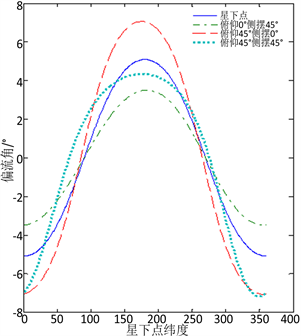Figure 8. Image shift speed and drift angle at different attitude angles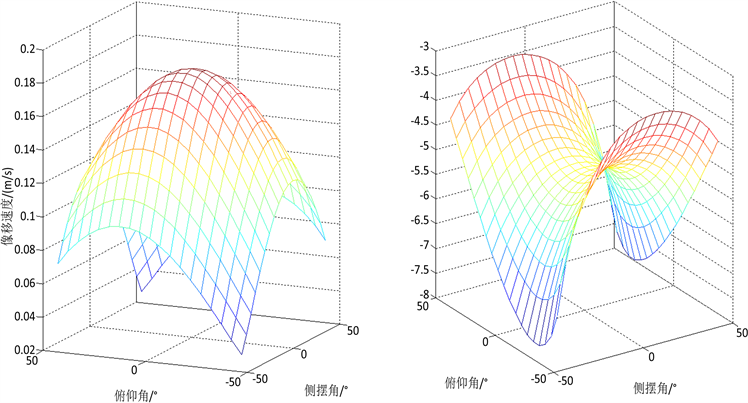Figure 9. Image shift speed & deviation angle vs. attitude angle diagram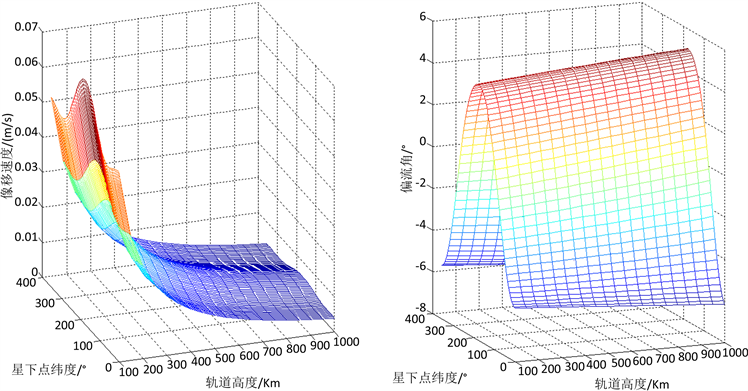Figure 10. Image shift speed & deviation angle vs. height

4. 结束语

 Miller, B. and Rubinovich, E. (2007) Image Motion Compensation at Charge-Coupled Device Photographing in Delay-Integration Mode. Information Protsessy, 7, 45-54.

 葛玉君, 赵健, 杨芳. 高分辨率光学遥感卫星平台技术综述[J]. 国际太空, 2013(5): 2-8.

 袁孝康. 星载TDICCD推扫相机的偏流角和速高比的计算模型[J]. 航天器工程, 2010, 19(1): 36-40.

 张媛. 星载TDI相机侧摆成像像移速度建模与分析[D]: [硕士学位论文]. 哈尔滨: 哈尔滨工业, 2013.

 黄群东, 杨芳, 赵键. 姿态对地指向不断变化成像时的像移速度计算[J]. 光学精密工程, 2012, 12(20): 2812-2820.

 李永昌. 离轴三反大视场空间相机像移速度场模型[J]. 红外与激光工程, 2016, 5(45): 0531001-0531007.

Top